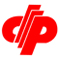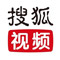竞 彩:英超天王山之战 中岸水手作客大败收场

所有彩票开奖查询

var urodz= new Date('10/05/2008 18:30'); var now = new Date(); var ile = urodz.getTime() - now.getTime(); var day = Math.floor(ile / (1000 * 60 * 60 * 24)); var dni = Math.floor(ile / (1000 * 60 * 60))-(24*day); var today = now.getFullYear(); var temp = ""; var wd; if(now.getDay()==0) wd = "日"; if(now.getDay()==1) wd = "一"; if(now.getDay()==2) wd = "二"; if(now.getDay()==3) wd = "三"; if(now.getDay()==4) wd = "四"; if(now.getDay()==5) wd = "五"; if(now.getDay()==6) wd = "六"; temp="购彩提醒.今天是:<span>"+today+"</span>年<span>"+(now。getMonth()+1)+"</span>月<span>"+now。getDate()+"</span>日"; temp+=" 星期"+wd+"<br>"; if (day>=0&&dni>=0){ temp+="距离<font color=blue>足彩08069期</font>截止<br>还有 <b><font color=red>"+day+"天 "+dni+"小时 </font></b>"}; document.write(temp);

• 福彩开奖直播
• 福彩演播室
•福彩开奖 每日21:15视频直播
•福彩演播室 每周日21:05首播彩票论坛精华

 中国福利彩票 中国体育彩票福彩直播开奖视频
捕鱼棋牌 休闲棋牌 电玩棋牌 蔚蓝棋牌 陕西棋牌 大富豪棋牌 左右棋牌 大菠萝棋牌 名门棋牌 其乐棋牌